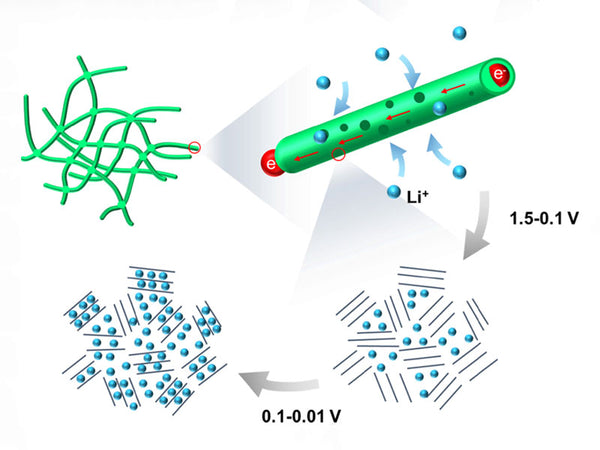Lithium ions are intercalated in the positive and negative electrodes according to the following steps: the transfer of lithium ions in the solution; the diffusion of lithium ions from the solution to the interface; the migration of lithium ions through the surface film; the transfer of charge; diffusion of lithium ions in the solid phase; accumulation of lithium ions in the solid phase (capacitive behavior). De-embedding is the opposite. The equivalent circuit describing these processes is a series of elements representing these steps.

When building a model, start with the simplest Randles circuit. Randles equivalent circuit is shown in Figure 1. Rs is the solution resistance, Cdl is the electric double layer capacitance, Rct is the charge transfer resistance, and ZW is the Warburg impedance of the reaction diffusion process.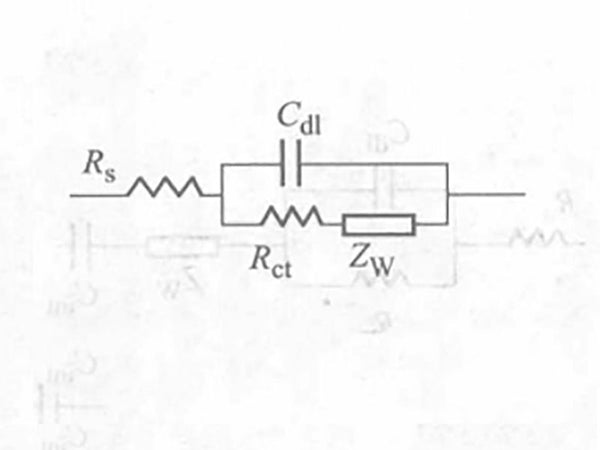Figure 1 - Randles equivalent circuit

Different processes can be easily distinguished in the complex impedance diagram: the high frequency is a semicircle with the origin on the real axis, from which Rs, Cdl and Rct can be obtained, and the low frequency part is a straight line at 45° to the real axis, whereby the diffusion coefficient can be obtained. For a finite-length diffusion, the phase angle increases as the frequency decreases, and the resistance reaches a limit value. In actual results, it is difficult to obtain a semicircle and a straight line of 45°. For this reason, the constant phase angle component CPE shown in Figure 2 can be introduced.Figure 2 - Equivalent circuit with constant phase angle components

Using this model, the kinetic parameters such as the exchange current density and diffusion coefficient of lithium ions in lithium manganese oxide can be obtained. Obviously, because the physical meaning of the constant phase angle element is extremely unclear, it is difficult for the constant phase angle element model to clearly describe the electrode process of lithium ion insertion and removal.

|K.Dokko et al. studied the AC impedance spectrum of a single LiCoO2 particle intercalating and desorbing lithium ions, and found that in most cases, the impedance plan is very similar to the results of the Randles model, except for differences at low frequencies. The obtained AC impedance spectrum is divided into three regions: a semicircle in the high-frequency region, the Warburg behavior in the low-frequency region, and the capacitive behavior in the lower frequency region. A modified Randles model is proposed (Figure 3).

In Figure 3, Cint is the capacitance of Li+ embedded in the active material, and the physical meaning of other components is the same as in Fig. 1. From the model fitting experiment results shown in Figure 3, the Rct, Cdl, Cint and other parameters of Li+ embedded in LiCoO2 were obtained. Someone was unable to fit the results obtained at 3.40~3.80V during the fitting process, and the resulting impedance spectra failed to explain the presence or absence of the surface film of the active material.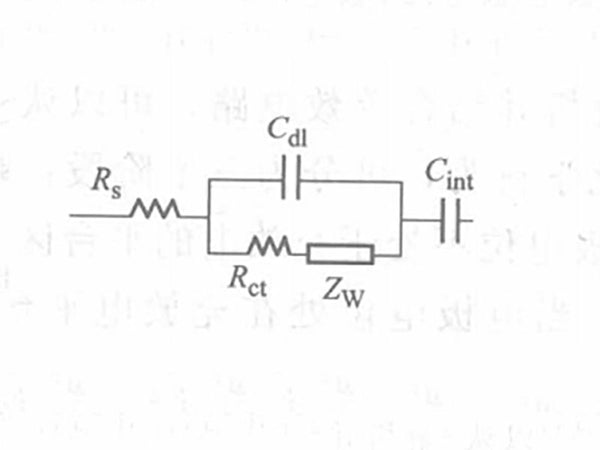Figure 3 - Modified Randles model

M.D. Levi et al. borrowed the equivalent circuit of the adsorption model shown in Figure 4 when looking for a suitable impedance model for lithium ion insertion and removal on the negative electrode carbon. The resulting impedance map is a deformed semicircle controlled by the dynamics in the high frequency zone and a straight line perpendicular to the real axis controlled by the thermodynamics in the low frequency zone (the physical meaning of each element in the figure is the same as in Figure 3).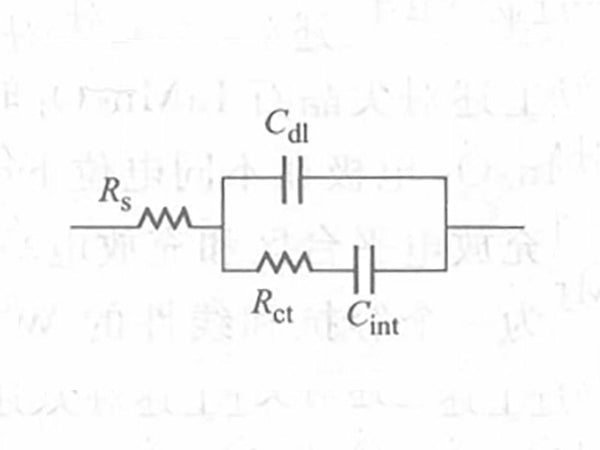Figure 4 - Adsorption equivalent circuit

No matter it is the negative electrode carbon or the positive transition metal oxide, there is a surface film on the surface of the active material. This surface film may be Li2CO3 or LiF formed by the decomposition of electrolyte. The surface film formed on the surface of the carbon negative electrode is similar to the surface film formed on the surface of metal lithium, and the impedance spectrum is shown as a flattened semicircle in the frequency range of 100kHz to several hertz. This spectrum reflects the migration process of lithium ions in the film. Due to the possible structure and composition of the surface layer, the equivalent circuit is the series connection of multiple R and C shown in Figure 5. Using Figure 5 to simulate the experimental results, the experimental spectrum is quite consistent with the fitted spectrum.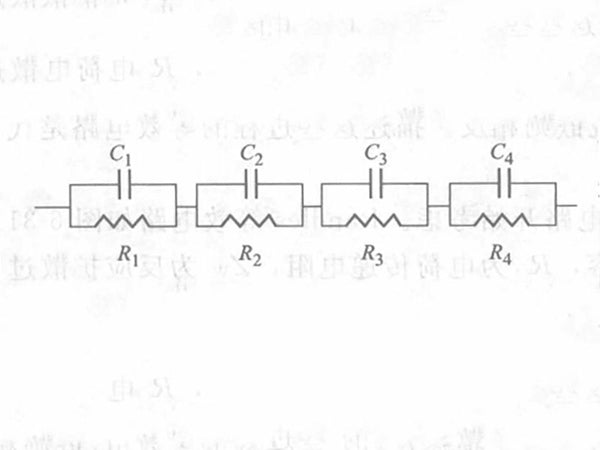Figure 5 - Voigt type equivalent circuit

Figure 6 shows the equivalent circuit describing the adsorption of organic molecules on the mercury electrode, which is called the FMG model (Frumkin and Mckik Gaykazyan), where Ra is the resistance describing the adsorption process. Based on this, M.D. Levi et al. proposed an equivalent circuit describing the insertion and removal of lithium ions in the positive and negative electrodes (Figure 7). Since diffusion occurs in the solid after the interface electron exchange, the Warburg impedance reflecting the diffusion should be placed in series with Cdl/Rct.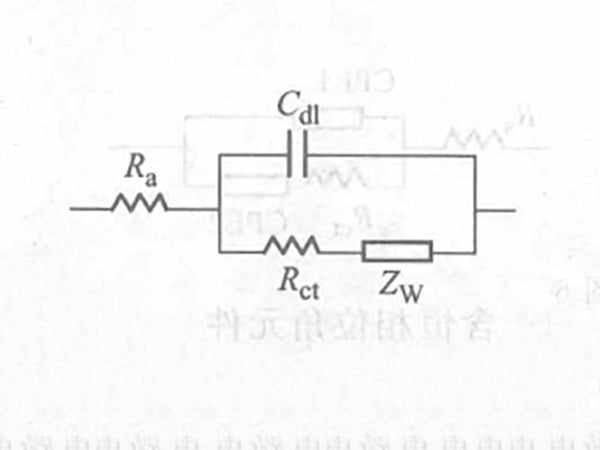Figure 6 - FMG equivalent circuitFigure 7 - Modified FMG equivalent circuit

Each step of insertion and extraction of lithium ions in the positive and negative electrodes can be described by corresponding electronic components. The equivalent electronic components of the steps performed at the same time are connected in parallel, and those that occur one after the other are connected in series. Because the diffusion of lithium ions in the solution is much easier than the diffusion in the solid, the diffusion step in the solution can be ignored. The conductivity of the solution is expressed by the solution resistance Rs, the migration of lithium ions in the surface film is described by C//R, the charge transfer of lithium ions at the interface between the surface film and the active material is described by Cdl//Rct, the diffusion of ions in the solid is described by ZW, and the accumulation and consumption of lithium ions in the solid are described by Cint. The desorption process of lithium ions in the positive and negative active materials of lithium-ion batteries can be fully described by the equivalent circuit shown in Figure 8, where Ci//Ri represents a multilayer film.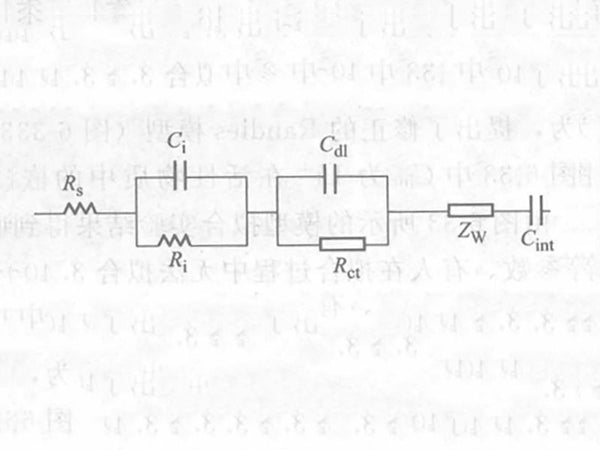Figure 8 - Modified Voigt-FMG equivalent circuit

The equivalent circuit shown in Figure 8 can effectively fit the experimental results. Impedance spectroscopy shows three areas: the high frequency area reflecting the diffusion of lithium ions in the surface film; the medium and high frequency area where the reactive film and the active material interface transfer, and the low frequency area where the reactive lithium ions accumulate and consume in the active material. The modified Voigt-FMG model that fully reacts lithium ions in the positive and negative electrodes of lithium-ion batteries can be used to facilitate the study of various factors affecting battery performance, find the reasons for battery capacity degradation and failure, and screen high-quality battery materials.